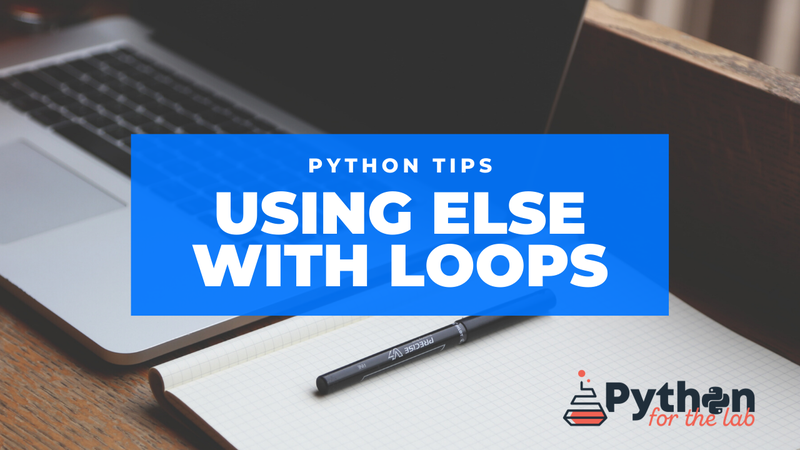#### Get all the information directly to your inbox

Get relevant information, unsubscribe at any time.# Python Tip: Using Else with Loops

### Learn how to use the else block with for and while loops

May 25, 2020 loops else for while tips

Most likely, you are aware of how to use the `else` statement with an `if` clause. However, Python also allows us to use them with loops. They are straightforward to understand and open some exciting possibilities. Before continuing, remember that `else` in this context should be called `no-break`.

Let's quickly see how a for-loop works:

``````start = 0
end = 10

for i in range(start, end):
print(i)
else:
print('End')``````

The code above will print the numbers from `0` to `9` and the `End` string. So far, nothing impressive, but check this out:

``````start = 0
end = 10
break_point = 5

for i in range(start, end):
print(i)
if i == break_point:
break
else:
print('Nothing')``````

The output will be all the numbers from `0` to `5`, but no string at the end. Now you can understand why it was called `no-break`. The same approach also works for `while` loops:

``````start = 0
end = 10
break_point = 5

i = start

while i < end:
print(i)
i += 1
if i == break_point:
break
else:
print('Nothing')``````

The fair question is, when would you use this pattern. A clear situation is when you are looking for an element. For example, you may be looking for a specific line in a file, and want to raise an exception if not found:

``````key_line = 'Key Line'
f = open('file.dat', 'r')
for line in f:
if line == key_line:
break
else: